Type
Solution Manual
Book Title
Fundamentals of Investments: Valuation and Management 8th Edition
ISBN 13
978-1259720697

### 978-1259720697 Chapter 14 Solution Manual

January 2, 2020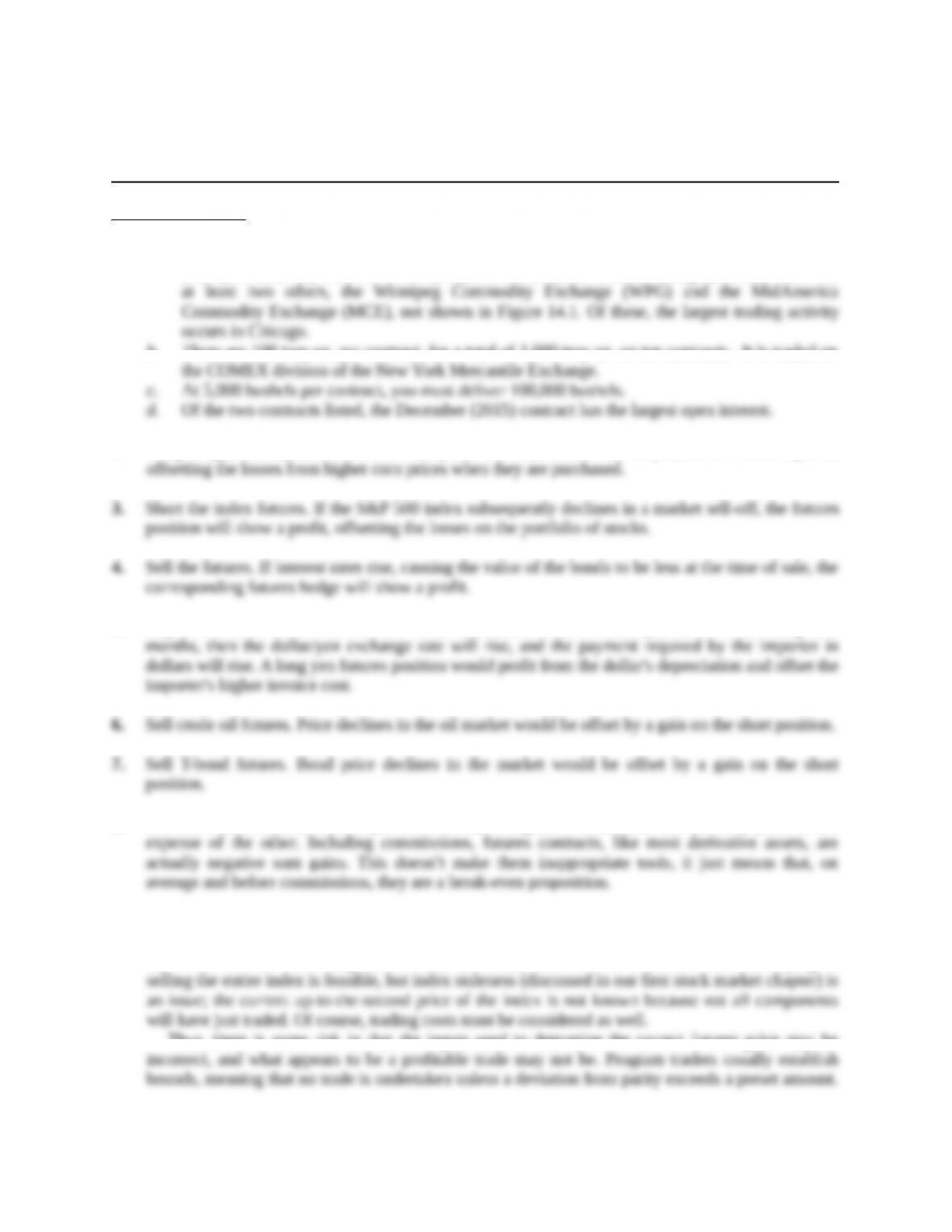Chapter 14
Futures Contracts
Concept Questions
1. a. Three are visible in Figure 14.1; wheat futures are traded on the Chicago Board of Trade
(CBT), Kansas City Board of Trade (KC), and Minneapolis Grain Exchange (MPLS). There are
b. There are 100 troy oz. per contract, for a total of 1,000 troy oz. on ten contracts. It is traded on
2. Long hedge; i.e., buy corn futures. If corn prices do rise, then the futures position will show a profit,
5. Buy yen futures. If the value of the dollar depreciates relative to the yen in the intervening four
8. It is true. Each contract has a buyer and a seller, a long and a short. One side can only profit at the
9. In reality, two factors make stock index arbitrage more difficult than it might appear. First, the
dividend yield on the index depends on the dividends that will be paid over the life of the contract;
this is not known with complete certainty and must, therefore, be estimated. Second, buying or
Thus, there is some risk in that the inputs used to determine the correct futures price may be
Education.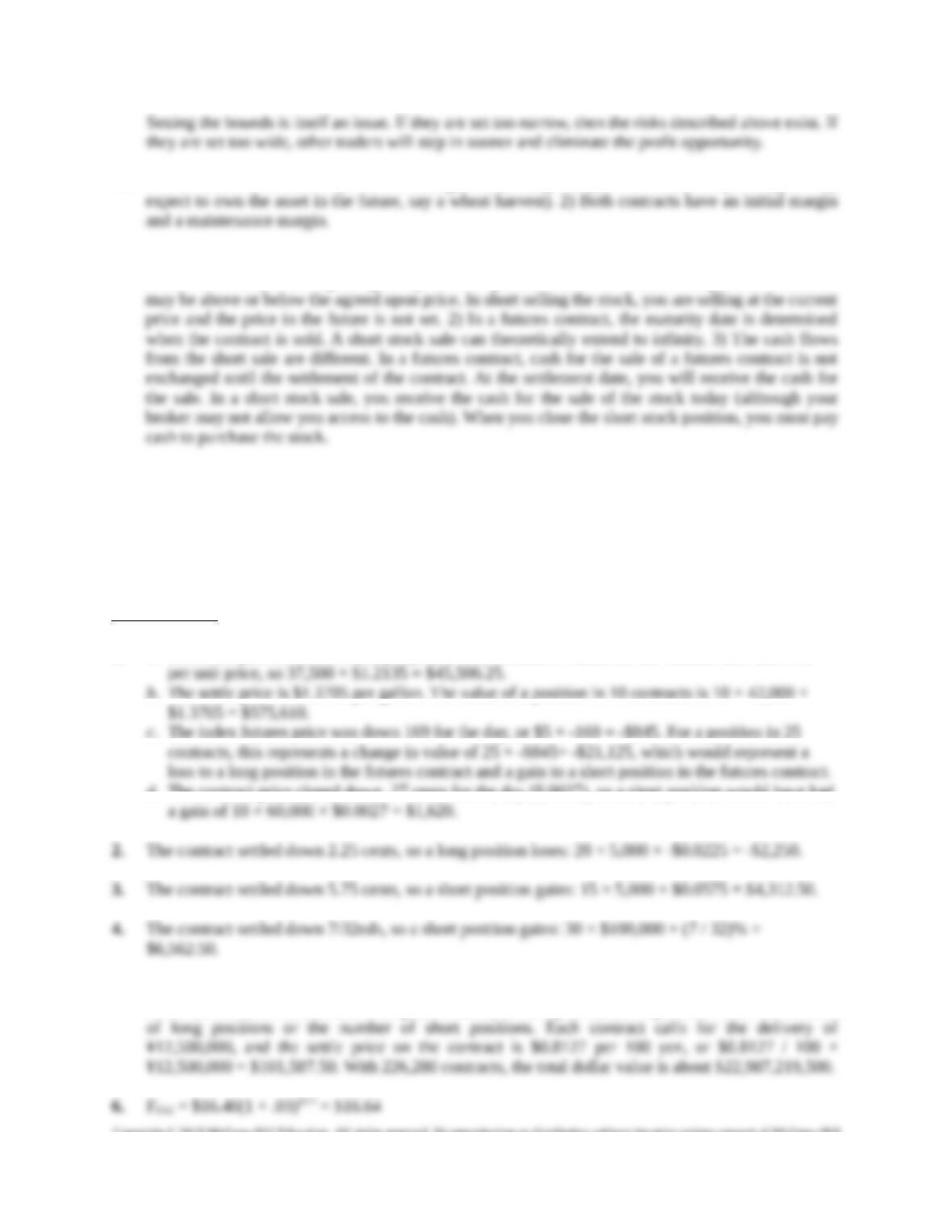10. There are two similarities. 1) You are selling an asset today that you do not currently own (you may
There are several major differences between a futures contract and short selling a stock. 1) With a
futures contract you are agreeing to a price at a specific date in the future. The price at settlement
Solutions to Questions and Problems
NOTE: All end of chapter problems were solved using a spreadsheet. Many problems require multiple
steps. Due to space and readability constraints, when these intermediate steps are included in this
solutions manual, rounding may appear to have occurred. However, the final answer for each problem is
found without rounding during any step in the problem.
Core Questions
1. a. The settle price is 121.35 cents per pound. One contract is valued as the contract size times the
b. The settle price is \$1.3705 per gallon. The value of a position in 10 contracts is 10 × 42,000 ×
c. The index futures price was down 169 for the day, or \$5 × -169 = -\$845. For a position in 25
d. The contract price closed down .27 cents for the day (\$.0027), so a short position would have had
5. The total open interest on the December 2015 Japanese Yen is 226,280 contracts. This is the number
of contracts. Each contract has a long and a short, so the open interest represents either the number
Education.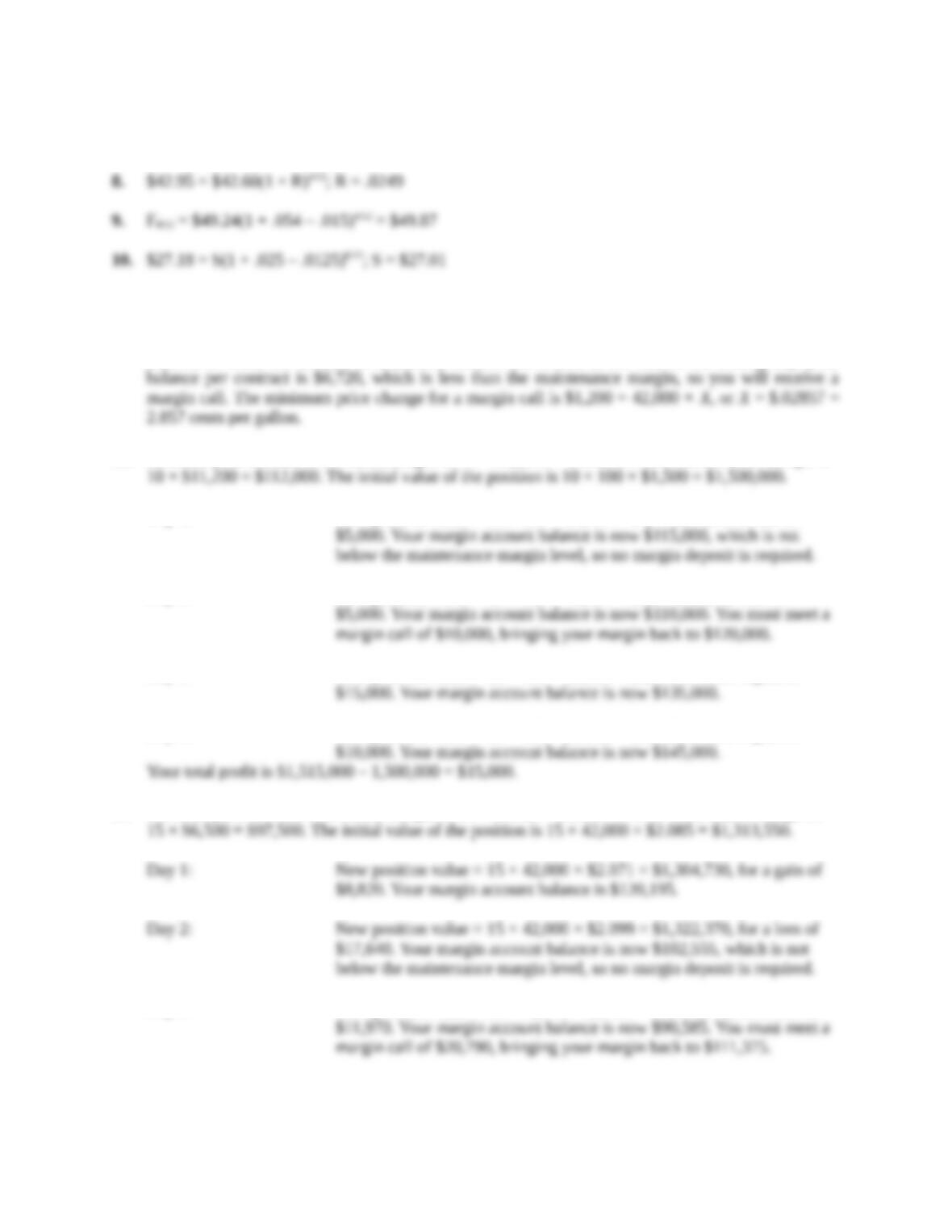7. \$94.90 = S(1 + .045)2/12; S = \$94.21
Intermediate Questions
11. If the contract settles down, a long position loses money. The loss per contract is: 42,000 × \$.04 =
\$1,680, so when the account is marked-to-market and settled at the end of the trading day, your
12. Establish your account at an initial margin of 10 × \$12,000 = \$120,000. Your maintenance margin is
Day 1: New position value = 10 × 100 × \$1,495 = \$1,495,000, for a loss of
Day 2: New position value = 10 × 100 × \$1,490 = \$1,490,000, for loss of
Day 3: New position value = 10 × 100 × \$1,505 = \$1,505,000, for a gain of
Day 4: New position value = 10 × 100 × \$1,515 = \$1,515,000, for a gain of
13. Establish your account at an initial margin of 15 × \$7,425 = \$111,375. Your maintenance margin is
Day 3: New position value = 15 × 42,000 × \$2.118 = \$1,334,340, for a loss of
Education.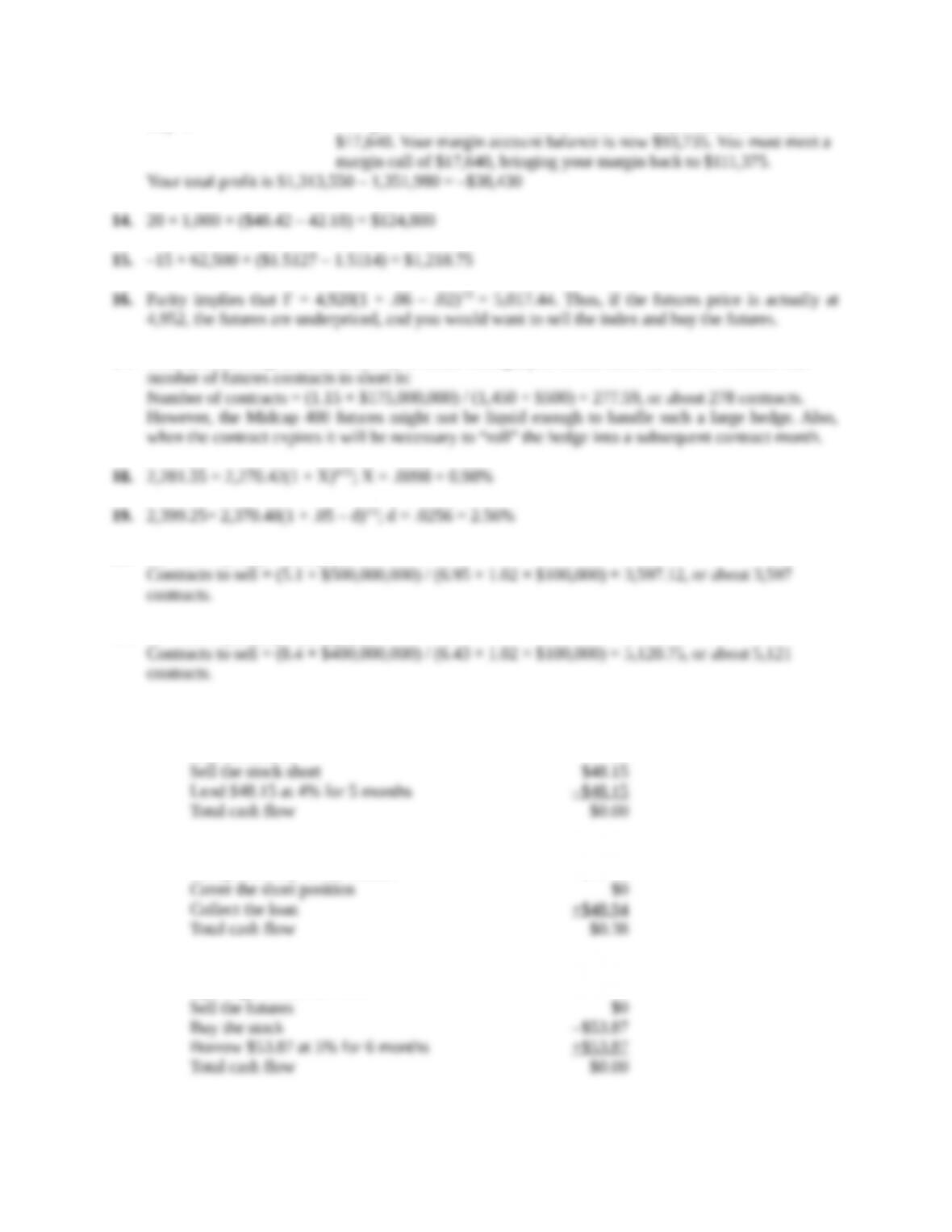Day 4: New position value = 15 × 42,000 × \$2.146 = \$1,351,980, for a loss of
17. Since you are long the asset (stocks), to create a hedge, you would short the futures contract. The
20. DF = 6.7 + (3/12) = 6.95 years
21. DF = 6.2 + (85/365) = 6.43 years
22. F = \$48.15(1 + .04)5/12 = \$48.94; the futures contract is underpriced
Opening transactions now:
Closing transactions:
Accept delivery on the futures –\$48.56
23. F = \$53.87(1 + .05)6/12 = \$55.20; the futures contract is overpriced
Opening transactions now:
Education.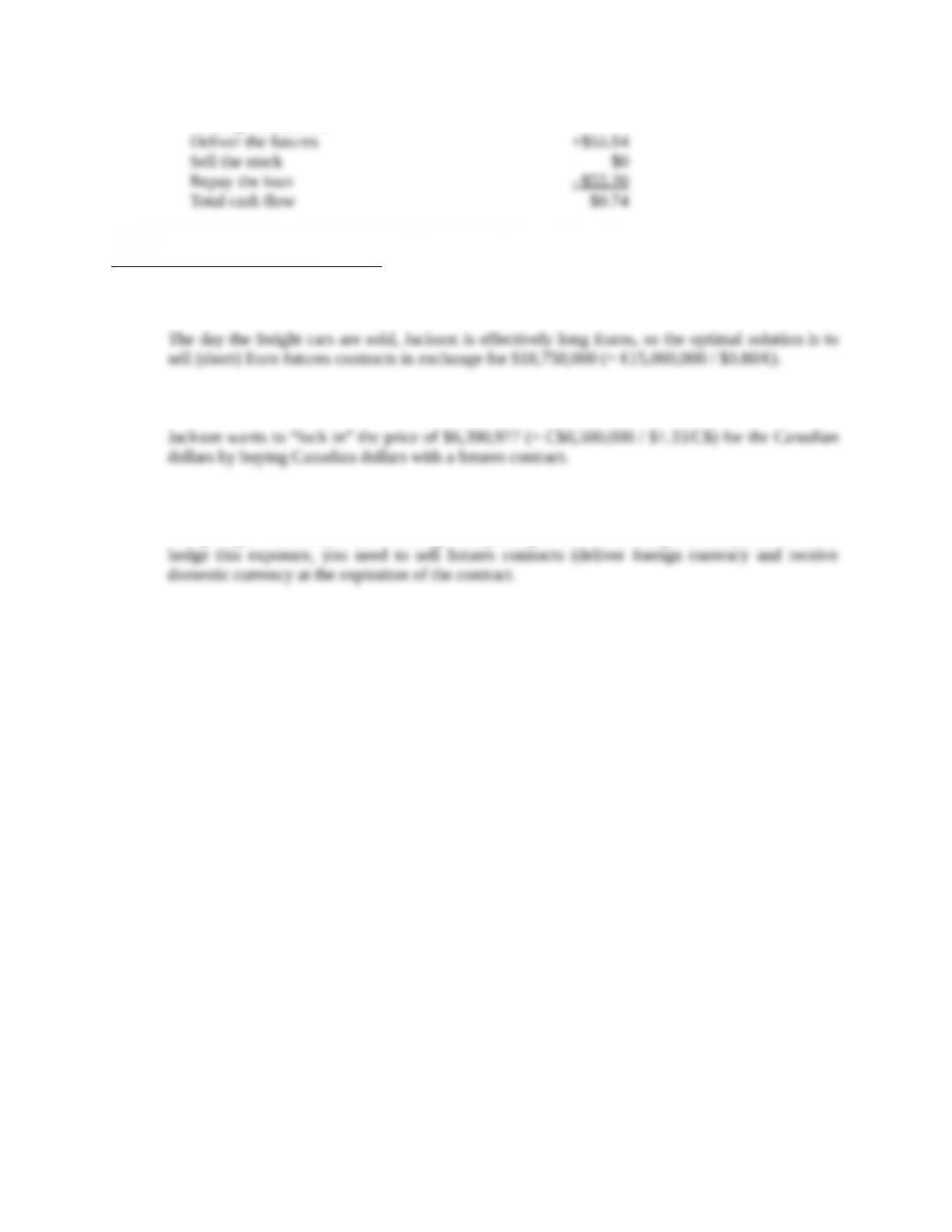Closing transactions:
CFA Exam Review by Kaplan Schweser
1. a
2. b
3. a
Being long the currency means holding or expecting to receive a foreign currency; therefore, to